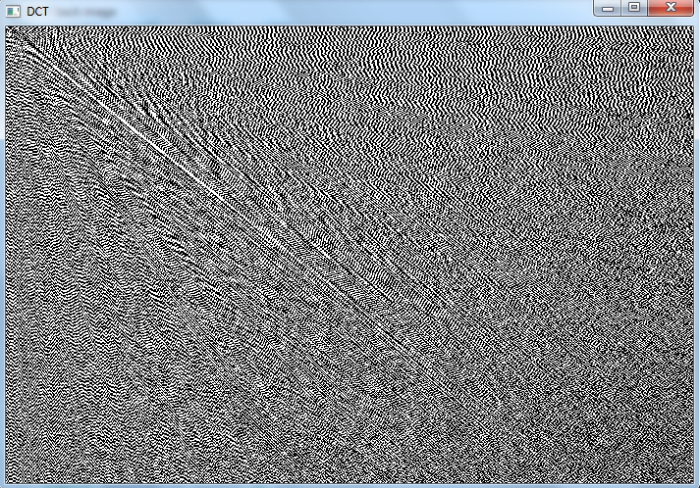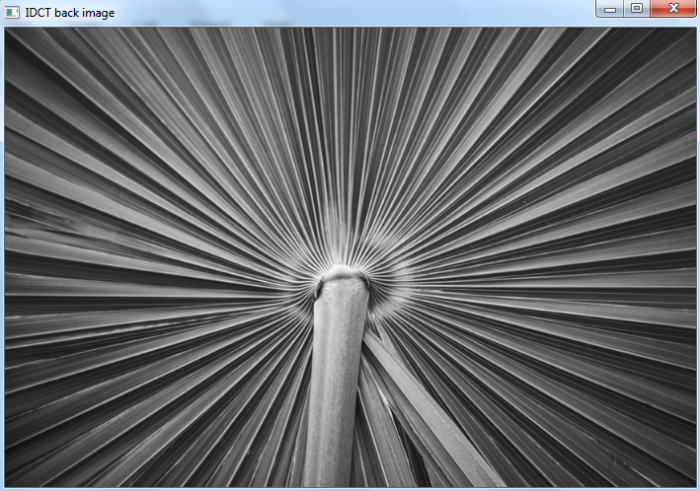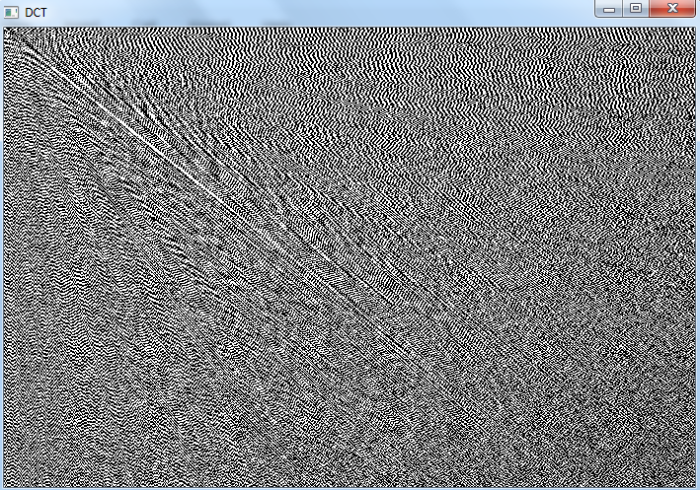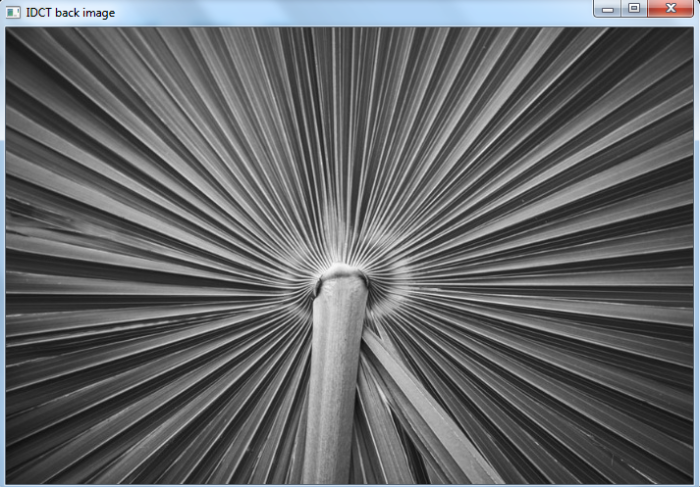# How to find discrete cosine transform of an image using OpenCV Python?

We apply cv2.dct() to find the discrete cosine transform of an image. This function transforms the grayscale image of dtype float32. It accepts two types of flag cv2.DCT_INVERSE or cv2.DCT_ROWS. To convert the transformed image to the original image we use cv2.idct().

## Steps

To find discrete cosine transform of an input image, you could follow the steps given below −

• Import the required libraries OpenCV and NumPy. Make sure you have already installed them.

• Read the input image using cv2.imread() method. Specify the full path of the image. Convert the input image to grayscale image using cv2.cvtColor() metod. Convert the grayscale image to np.float32.

• Find the discrete cosine transform of the image using cv2.dct(). This method takes a grayscale image in floating point. Pass flag cv2.DCT_INVERSE or cv2.DCT_ROWS to the cv2.dct() function. Visualize the discrete transform of the input image using cv2.imshow() method.

• To visualize the input image back after the discrete cosine transform, apply inverse discrete cosine transform cv2.idct(). And convert the image to np.uint8.

Let's see the examples to find discrete cosine transform of an input image.

## Input Image

We will use the below image as the input files in the examples below.## Example

In this example, we find discrete cosine transform of the input image. We pass flag cv2.DCT_INVERSE to the cv2.dct() function.

# import required libraries
import cv2
import numpy as np

# read input image as grayscale

# convert the grayscale to float32
imf = np.float32(img) # float conversion

# find discrete cosine transform
dst = cv2.dct(imf, cv2.DCT_INVERSE)

# apply inverse discrete cosine transform
img1 = cv2.idct(dst)

# convert to uint8
img1 = np.uint8(img)

# display the images
cv2.imshow("DCT", dst)
cv2.waitKey(0)
cv2.imshow("IDCT back image", img1)
cv2.waitKey(0)
cv2.destroyAllWindows()


## Output

When we run the above program, it will produce the following output windows −## Example

In this example, we find discrete cosine transform of the input image. We pass flag cv2.DCT_ROWS to the cv2.dct() function.

# import required libraries
import cv2
import matplotlib.pyplot as plt
import numpy as np

# read input image as grayscale

# convert the grayscale to float32
imf = np.float32(img) # float conversion

# find discrete cosine transform
dct = cv2.dct(imf, cv2.DCT_ROWS)

# apply inverse discrete cosine transform
img1 = cv2.idct(dct)

# convert to uint8
img1 = np.uint8(img)

# display the image
cv2.imshow("DCT", dct)
cv2.waitKey(0)
cv2.imshow("IDCT back image", img1)
cv2.waitKey(0)
cv2.destroyAllWindows()


## Output

When we run the above program, it will produce the following output windows −Updated on: 02-Dec-2022

4K+ Views